xIonization energyEncyclopedia
The ionization energy of a chemical species, i.e. an atom
Atom
The atom is a basic unit of matter that consists of a dense central nucleus surrounded by a cloud of negatively charged electrons. The atomic nucleus contains a mix of positively charged protons and electrically neutral neutrons...

or molecule
Molecule
A molecule is an electrically neutral group of at least two atoms held together by covalent chemical bonds. Molecules are distinguished from ions by their electrical charge...

, is the energy required to remove an electron from the species to a practically infinite distance. Large atoms or molecules have a low ionization energy, while small molecules tend to have higher ionization energies.

The property is alternately still often called the ionization potential, measured in volts. In chemistry
Chemistry
Chemistry is the science of matter, especially its chemical reactions, but also its composition, structure and properties. Chemistry is concerned with atoms and their interactions with other atoms, and particularly with the properties of chemical bonds....

it is often referred to one mole of substance (molar ionization energy or enthalpy) and reported in kJ/mol. In atomic physics the ionization energy is typically measured in the unit electron volt (eV).

The ionization energy is different for electrons of different atomic or molecular orbitals. More generally, the nth ionization energy is the energy required to strip off the nth electron after the first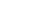electrons have been removed. It is considered a measure of the tendency of an atom or ion to surrender an electron, or the strength of the electron binding; the greater the ionization energy, the more difficult it is to remove an electron. The ionization energy may be an indicator of the reactivity of an element. Elements with a low ionization energy tend to be reducing agents and form cations, which in turn combine with anions to form salts.

Electron binding energy (BE), more accurately, is the energy
Energy
In physics, energy is an indirectly observed quantity. It is often understood as the ability a physical system has to do work on other physical systems...

required to release an electron from its atomic or molecular orbital when adsorbed to a surface rather than a free atom. Binding energy values are normally reported as positive values with units of eV
Electronvolt
In physics, the electron volt is a unit of energy equal to approximately joule . By definition, it is equal to the amount of kinetic energy gained by a single unbound electron when it accelerates through an electric potential difference of one volt...

. The binding energies of 1s electrons are roughly proportional to (Z-1)² (Moseley's law
Moseley's law
Moseley's law is an empirical law concerning the characteristic x-rays that are emitted by atoms. The law was discovered and published by the English physicist Henry Moseley in 1913...

).

## Values and trends

Main article: Molar ionization energies of the elements

Generally the (n+1)th ionization energy is larger than the nth ionization energy. Always, the next ionization energy involves removing an electron from an orbital closer to the nucleus. Electrons in the closer orbitals experience greater forces of electrostatic attraction; thus, their removal requires increasingly more energy. Ionization energy becomes greater up and to the right of the periodic table.

Some values for elements of the third period are given in the following table:
Successive molar ionization energies in kJ
Joule
The joule ; symbol J) is a derived unit of energy or work in the International System of Units. It is equal to the energy expended in applying a force of one newton through a distance of one metre , or in passing an electric current of one ampere through a resistance of one ohm for one second...

/mol
Mole (unit)
The mole is a unit of measurement used in chemistry to express amounts of a chemical substance, defined as an amount of a substance that contains as many elementary entities as there are atoms in 12 grams of pure carbon-12 , the isotope of carbon with atomic weight 12. This corresponds to a value...

(96.485 kJ/mol = 1 eV/particle)
Element First Second Third Fourth Fifth Sixth Seventh
Na
Sodium
Sodium is a chemical element with the symbol Na and atomic number 11. It is a soft, silvery-white, highly reactive metal and is a member of the alkali metals; its only stable isotope is 23Na. It is an abundant element that exists in numerous minerals, most commonly as sodium chloride...

496 4,560
Mg
Magnesium
Magnesium is a chemical element with the symbol Mg, atomic number 12, and common oxidation number +2. It is an alkaline earth metal and the eighth most abundant element in the Earth's crust and ninth in the known universe as a whole...

738 1,450 7,730
Al
Aluminium
Aluminium or aluminum is a silvery white member of the boron group of chemical elements. It has the symbol Al, and its atomic number is 13. It is not soluble in water under normal circumstances....

577 1,816 2,881 11,600
Si
Silicon
Silicon is a chemical element with the symbol Si and atomic number 14. A tetravalent metalloid, it is less reactive than its chemical analog carbon, the nonmetal directly above it in the periodic table, but more reactive than germanium, the metalloid directly below it in the table...

786 1,577 3,228 4,354 16,100
P
Phosphorus
Phosphorus is the chemical element that has the symbol P and atomic number 15. A multivalent nonmetal of the nitrogen group, phosphorus as a mineral is almost always present in its maximally oxidized state, as inorganic phosphate rocks...

1,060 1,890 2,905 4,950 6,270 21,200
S
Sulfur
Sulfur or sulphur is the chemical element with atomic number 16. In the periodic table it is represented by the symbol S. It is an abundant, multivalent non-metal. Under normal conditions, sulfur atoms form cyclic octatomic molecules with chemical formula S8. Elemental sulfur is a bright yellow...

999.6 2,260 3,375 4,565 6,950 8,490 27,107
Cl
Chlorine
Chlorine is the chemical element with atomic number 17 and symbol Cl. It is the second lightest halogen, found in the periodic table in group 17. The element forms diatomic molecules under standard conditions, called dichlorine...

1,256 2,295 3,850 5,160 6,560 9,360 11,000
Ar
Argon
Argon is a chemical element represented by the symbol Ar. Argon has atomic number 18 and is the third element in group 18 of the periodic table . Argon is the third most common gas in the Earth's atmosphere, at 0.93%, making it more common than carbon dioxide...

1,520 2,665 3,945 5,770 7,230 8,780 12,000

Large jumps in the successive molar ionization energies occur when passing noble gas
Noble gas
The noble gases are a group of chemical elements with very similar properties: under standard conditions, they are all odorless, colorless, monatomic gases, with very low chemical reactivity...

configurations. For example, as can be seen in the table above, the first two molar ionization energies of magnesium (stripping the two 3s electrons from a magnesium atom) are much smaller than the third, which requires stripping off a 2p electron from the very stable neon
Neon
Neon is the chemical element that has the symbol Ne and an atomic number of 10. Although a very common element in the universe, it is rare on Earth. A colorless, inert noble gas under standard conditions, neon gives a distinct reddish-orange glow when used in either low-voltage neon glow lamps or...

configuration of Mg2+.
Ionization energy is also a periodic trend within the periodic table
Periodic table
The periodic table of the chemical elements is a tabular display of the 118 known chemical elements organized by selected properties of their atomic structures. Elements are presented by increasing atomic number, the number of protons in an atom's atomic nucleus...

organization. Moving left to right within a period or upward within a group, the first ionization energy generally increases. As the atomic radius
The atomic radius of a chemical element is a measure of the size of its atoms, usually the mean or typical distance from the nucleus to the boundary of the surrounding cloud of electrons...

decreases, it becomes harder to remove an electron that is closer to a more positively charged nucleus.
ionization enthalpy increases from left to right in a period and decreases from top to bottom in a group.

## Electrostatic explanation

Atomic ionization energy can be predicted by an analysis using electrostatic potential and the Bohr model
Bohr model
In atomic physics, the Bohr model, introduced by Niels Bohr in 1913, depicts the atom as a small, positively charged nucleus surrounded by electrons that travel in circular orbits around the nucleus—similar in structure to the solar system, but with electrostatic forces providing attraction,...

of the atom, as follows.

Consider an electron of charge -e
Elementary charge
The elementary charge, usually denoted as e, is the electric charge carried by a single proton, or equivalently, the absolute value of the electric charge carried by a single electron. This elementary charge is a fundamental physical constant. To avoid confusion over its sign, e is sometimes called...

and an atomic nucleus with charge +Ze, where Z is the number of protons in the nucleus. According to the Bohr model
Bohr model
In atomic physics, the Bohr model, introduced by Niels Bohr in 1913, depicts the atom as a small, positively charged nucleus surrounded by electrons that travel in circular orbits around the nucleus—similar in structure to the solar system, but with electrostatic forces providing attraction,...

, if the electron were to approach and bind with the atom, it would come to rest at a certain radius a. The electrostatic potential V at distance a from the ionic nucleus, referenced to a point infinitely far away, is: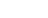Since the electron is negatively charged, it is drawn inwards by this positive electrostatic potential. The energy required for the electron to "climb out" and leave the atom is: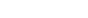This analysis is incomplete, as it leaves the distance a as an unknown variable. It can be made more rigorous by assigning to each electron of every chemical element
Chemical element
A chemical element is a pure chemical substance consisting of one type of atom distinguished by its atomic number, which is the number of protons in its nucleus. Familiar examples of elements include carbon, oxygen, aluminum, iron, copper, gold, mercury, and lead.As of November 2011, 118 elements...

a characteristic distance, chosen so that this relation agrees with experimental data.

It is possible to expand this model considerably by taking a semi-classical approach, in which momentum is quantized. This approach works very well for the hydrogen atom, which only has one electron. The magnitude of the angular momentum for a circular orbit is: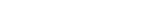The total energy of the atom is the sum of the kinetic and potential energies, that is: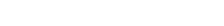Velocity can be eliminated from the kinetic energy term by setting the Coulomb attraction equal to the centripetal force, giving: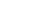Solving the angular momentum for v and substituting this into the expression for kinetic energy, we have: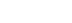This establishes the dependence of the radius on n. That is: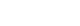Now the energy can be found in terms of Z, e, and r. Using the new value for the kinetic energy in the total energy equation above, it is found that: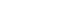At its smallest value, n is equal to 1 and r is the Bohr radius
The Bohr radius is a physical constant, approximately equal to the most probable distance between the proton and electron in a hydrogen atom in its ground state. It is named after Niels Bohr, due to its role in the Bohr model of an atom...

a0. Now, the equation for the energy can be established in terms of the Bohr radius. Doing so gives the result: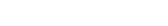## Quantum-mechanical explanation

According to the more complete theory of quantum mechanics
Quantum mechanics
Quantum mechanics, also known as quantum physics or quantum theory, is a branch of physics providing a mathematical description of much of the dual particle-like and wave-like behavior and interactions of energy and matter. It departs from classical mechanics primarily at the atomic and subatomic...

, the location of an electron is best described as a probability distribution. The energy can be calculated by integrating over this cloud. The cloud's underlying mathematical representation is the wavefunction
Wavefunction
Not to be confused with the related concept of the Wave equationA wave function or wavefunction is a probability amplitude in quantum mechanics describing the quantum state of a particle and how it behaves. Typically, its values are complex numbers and, for a single particle, it is a function of...

which is built from Slater determinant
Slater determinant
In quantum mechanics, a Slater determinant is an expression that describes the wavefunction of a multi-fermionic system that satisfies anti-symmetry requirements and consequently the Pauli exclusion principle by changing sign upon exchange of fermions . It is named for its discoverer, John C...

s consisting of molecular spin orbitals. These are related by Pauli's exclusion principle to the antisymmetrized products of the atomic
Atomic orbital
An atomic orbital is a mathematical function that describes the wave-like behavior of either one electron or a pair of electrons in an atom. This function can be used to calculate the probability of finding any electron of an atom in any specific region around the atom's nucleus...

or molecular orbitals.

In general, calculating the nth ionization energy requires calculating the energies of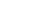and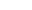electron systems. Calculating these energies exactly is not possible except for the simplest systems (i.e. hydrogen), primarily because of difficulties in integrating the correlation terms. Therefore, approximation methods are routinely employed, with different methods varying in complexity (computational time) and in accuracy compared to empirical data. This has become a well-studied problem and is routinely done in computational chemistry
Computational chemistry
Computational chemistry is a branch of chemistry that uses principles of computer science to assist in solving chemical problems. It uses the results of theoretical chemistry, incorporated into efficient computer programs, to calculate the structures and properties of molecules and solids...

. At the lowest level of approximation, the ionization energy is provided by Koopmans' theorem
Koopmans' theorem
Koopmans' theorem states that in closed-shell Hartree-Fock theory, the first ionization energy of a molecular system is equal to the negative of the orbital energy of the highest occupied molecular orbital...

.

## Vertical and adiabatic ionization energy in molecules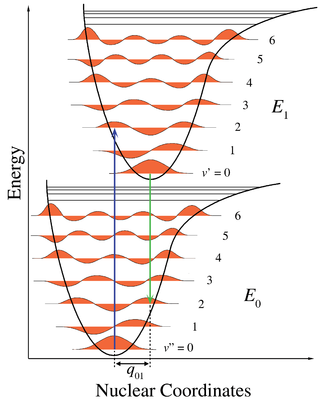Ionization of molecules often leads to changes in molecular geometry
Molecular geometry
Molecular geometry or molecular structure is the three-dimensional arrangement of the atoms that constitute a molecule. It determines several properties of a substance including its reactivity, polarity, phase of matter, color, magnetism, and biological activity.- Molecular geometry determination...

, and two types of (first) ionization energy are defined – adiabatic and vertical. The adiabatic
The adiabatic theorem is an important concept in quantum mechanics. Its original form, due to Max Born and Vladimir Fock , can be stated as follows:...

ionization energy of a molecule is the difference between the energies of the neutral molecule and its positive ion, each in its vibrational
Molecular vibration
A molecular vibration occurs when atoms in a molecule are in periodic motion while the molecule as a whole has constant translational and rotational motion...

ground state
Ground state
The ground state of a quantum mechanical system is its lowest-energy state; the energy of the ground state is known as the zero-point energy of the system. An excited state is any state with energy greater than the ground state...

, and possibly with different equilibrium geometries.

The vertical ionization energy is the energy required to form a positive ion with the same geometry as the neutral molecule, corresponding to a vertical transition on a diagram of potential energy as a function of geometry. Such ionization is often accompanied by vibrational excitation. According to the Franck-Condon principle
Franck-Condon principle
The Franck–Condon principle is a rule in spectroscopy and quantum chemistry that explains the intensity of vibronic transitions. Vibronic transitions are the simultaneous changes in electronic and vibrational energy levels of a molecule due to the absorption or emission of a photon of the...

, vertical ionization is the most probable, since electrons move much more rapidly than nuclei and the ionized electron departs before the nuclei have time to adjust their position.

For a diatomic molecule the geometry is defined by one bond length
Bond length
- Explanation :Bond length is related to bond order, when more electrons participate in bond formation the bond will get shorter. Bond length is also inversely related to bond strength and the bond dissociation energy, as a stronger bond will be shorter...

. The removal of an electron from a bonding molecular orbital
Molecular orbital
In chemistry, a molecular orbital is a mathematical function describing the wave-like behavior of an electron in a molecule. This function can be used to calculate chemical and physical properties such as the probability of finding an electron in any specific region. The term "orbital" was first...

weakens the bond and increases the bond length. In Figure 1, the lower potential energy curve
Potential energy surface
A potential energy surface is generally used within the adiabatic or Born–Oppenheimer approximation in quantum mechanics and statistical mechanics to model chemical reactions and interactions in simple chemical and physical systems...

is for the neutral molecule as a function of bond length. The horizontal lines are vibrational levels
Molecular vibration
A molecular vibration occurs when atoms in a molecule are in periodic motion while the molecule as a whole has constant translational and rotational motion...

with their associated vibrational wave functions
Quantum harmonic oscillator
The quantum harmonic oscillator is the quantum-mechanical analog of the classical harmonic oscillator. Because an arbitrary potential can be approximated as a harmonic potential at the vicinity of a stable equilibrium point, it is one of the most important model systems in quantum mechanics...

. The upper curve is the ionized state, with its minimum energy further to the right since the bond length in the ion is greater. The adiabatic ionization is the diagonal transition to the vibrational ground state of the ion. Vertical ionization involves vibrational excitation of the ionic state and therefore requires greater energy.

• Bragg-Gray Cavity Theory
Bragg-Gray Cavity Theory
According to the Bragg–Gray cavity theory, the ionization produced within a gas-filled cavity inside a medium is related to the energy absorbed in that surrounding medium....

• Electronegativity
Electronegativity
Electronegativity, symbol χ , is a chemical property that describes the tendency of an atom or a functional group to attract electrons towards itself. An atom's electronegativity is affected by both its atomic number and the distance that its valence electrons reside from the charged nucleus...

• Ionization
Ionization
Ionization is the process of converting an atom or molecule into an ion by adding or removing charged particles such as electrons or other ions. This is often confused with dissociation. A substance may dissociate without necessarily producing ions. As an example, the molecules of table sugar...

• The work function
Work function
In solid-state physics, the work function is the minimum energy needed to remove an electron from a solid to a point immediately outside the solid surface...

is the energy required to strip an electron from a solid
Solid
Solid is one of the three classical states of matter . It is characterized by structural rigidity and resistance to changes of shape or volume. Unlike a liquid, a solid object does not flow to take on the shape of its container, nor does it expand to fill the entire volume available to it like a...

.
• Ion
Ion
An ion is an atom or molecule in which the total number of electrons is not equal to the total number of protons, giving it a net positive or negative electrical charge. The name was given by physicist Michael Faraday for the substances that allow a current to pass between electrodes in a...

• Koopmans' theorem
Koopmans' theorem
Koopmans' theorem states that in closed-shell Hartree-Fock theory, the first ionization energy of a molecular system is equal to the negative of the orbital energy of the highest occupied molecular orbital...

• Di-tungsten tetra(hpp)
Di-tungsten tetra(hpp)
Di-tungsten tetra is the name of the coordination compound with the formula W24. This material consists of a pair of tungsten centers linked by the conjugate base of four hexahydropyrimidopyrimidine ligands...

has the lowest recorded ionization energy for a stable chemical compound
Chemical compound
A chemical compound is a pure chemical substance consisting of two or more different chemical elements that can be separated into simpler substances by chemical reactions. Chemical compounds have a unique and defined chemical structure; they consist of a fixed ratio of atoms that are held together...

.
• Electron affinity
Electron affinity
The Electron affinity of an atom or molecule is defined as the amount of energy released when an electron is added to a neutral atom or molecule to form a negative ion....

• Debye length
Debye length
In plasma physics, the Debye length , named after the Dutch physicist and physical chemist Peter Debye, is the scale over which mobile charge carriers screen out electric fields in plasmas and other conductors. In other words, the Debye length is the distance over which significant charge...